Anúncio

# Leverage nikunj

16 de Oct de 2011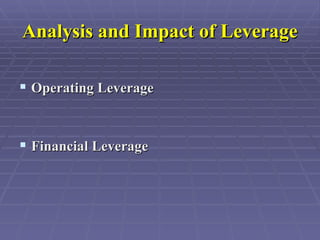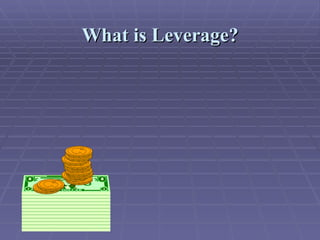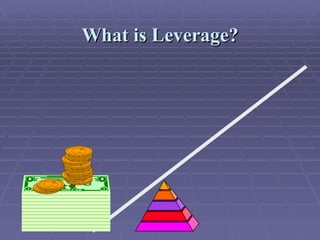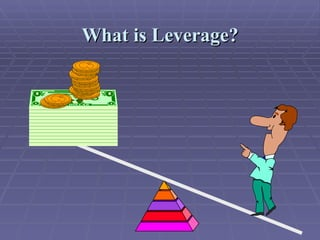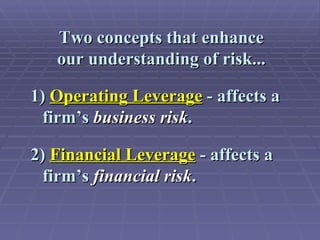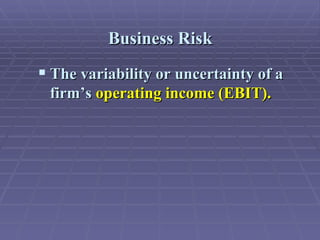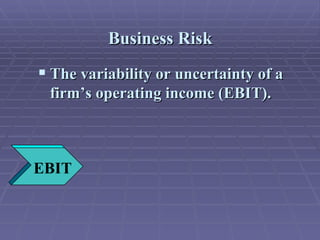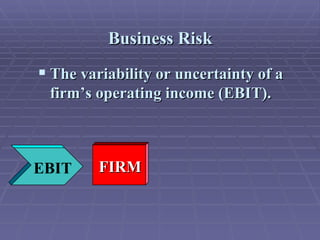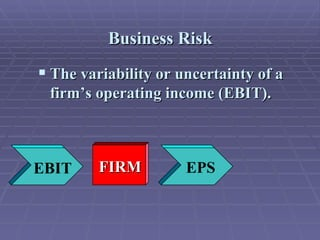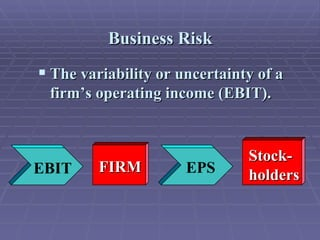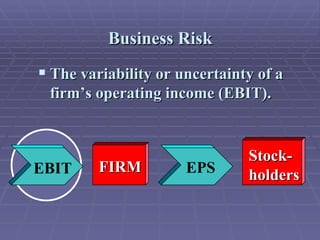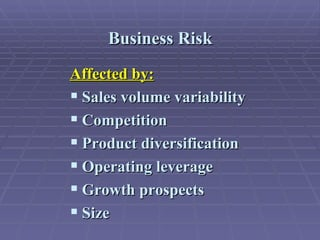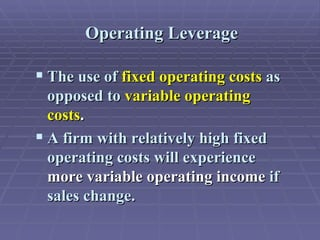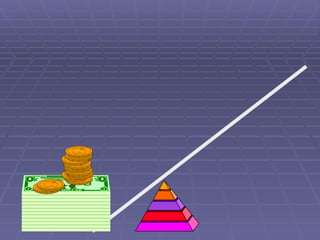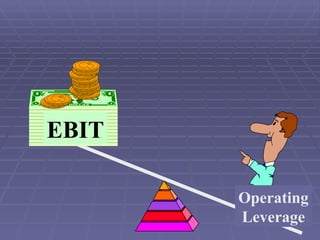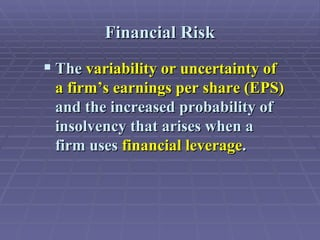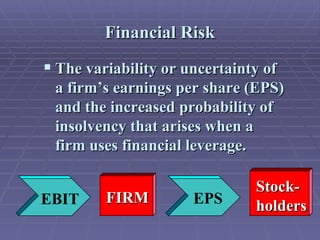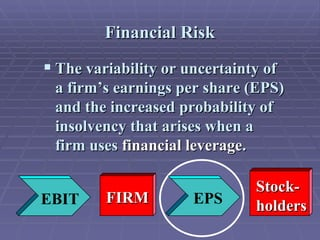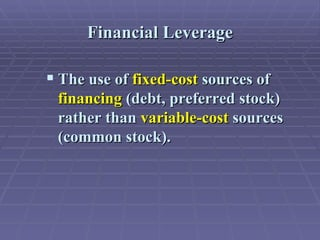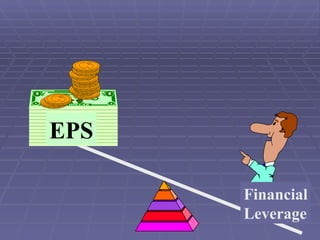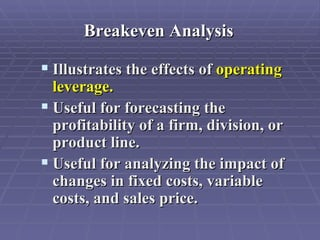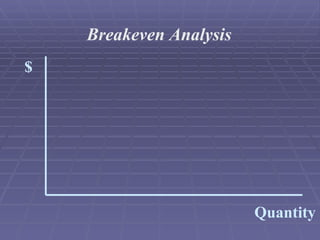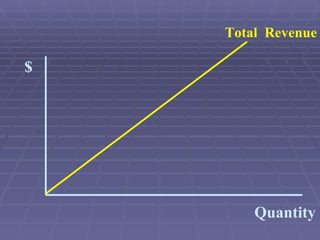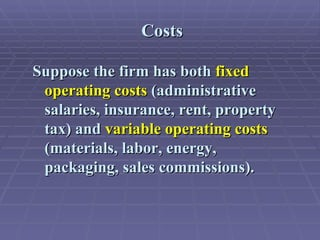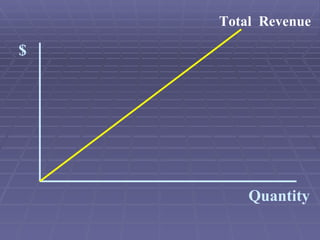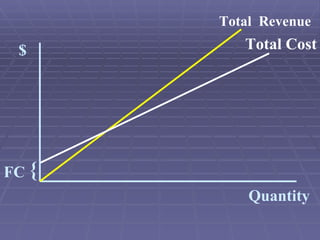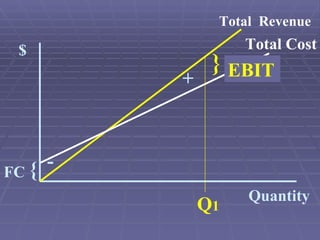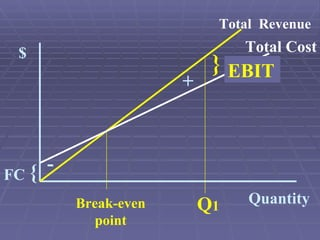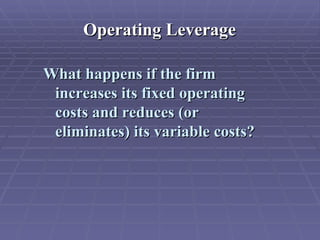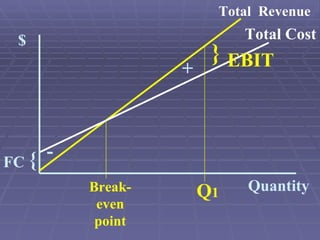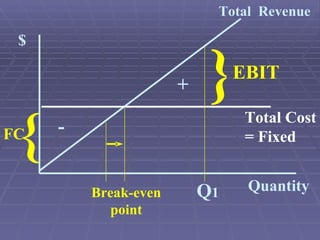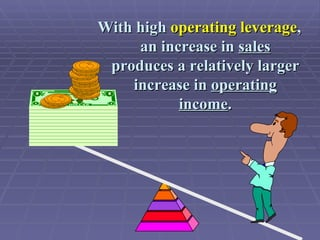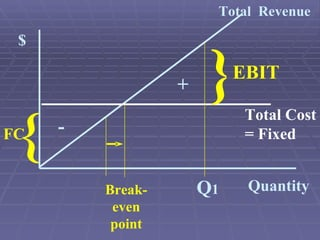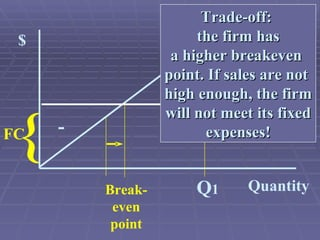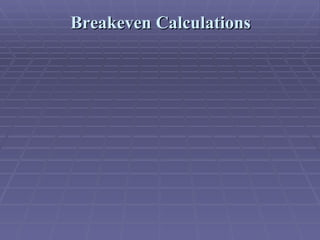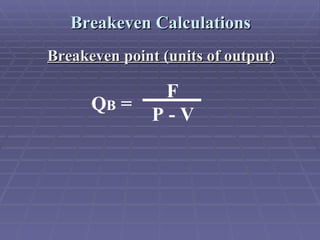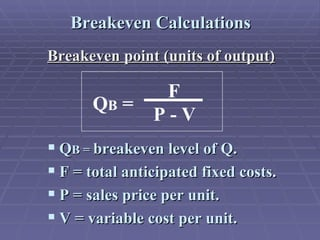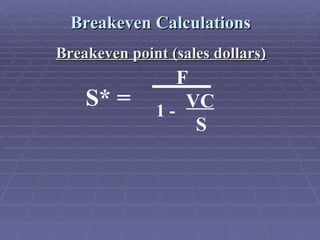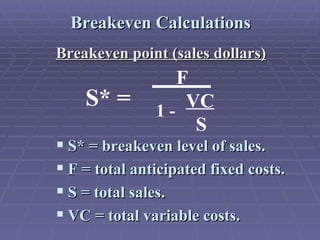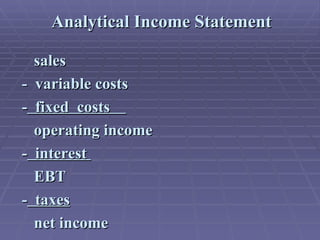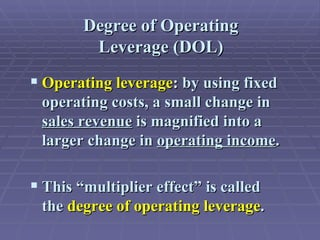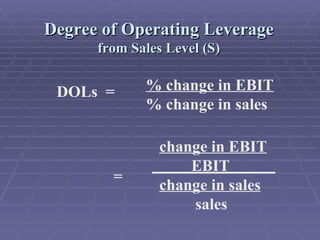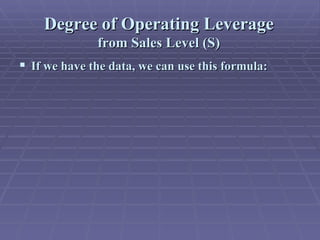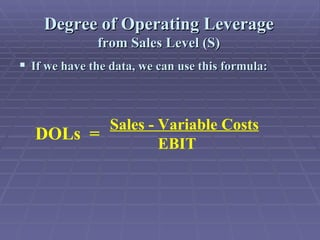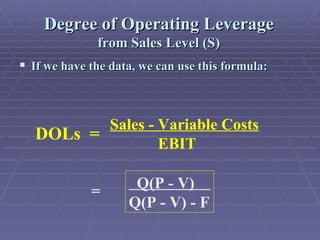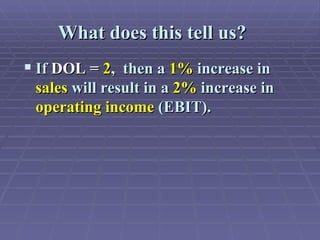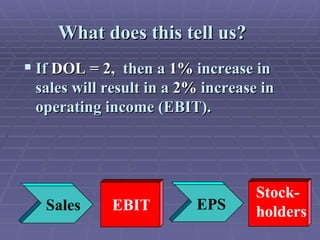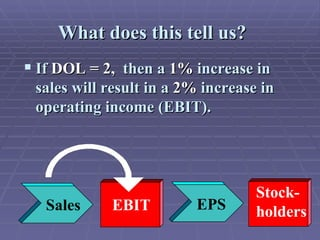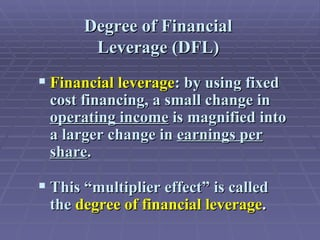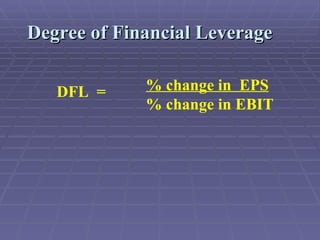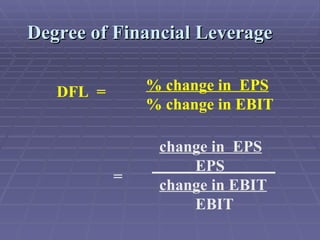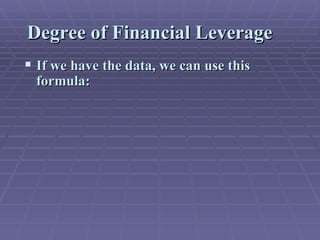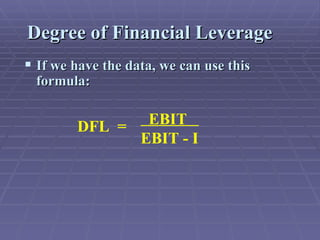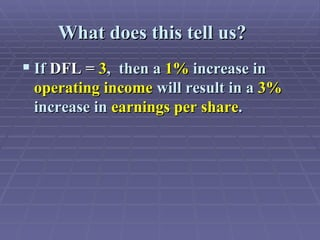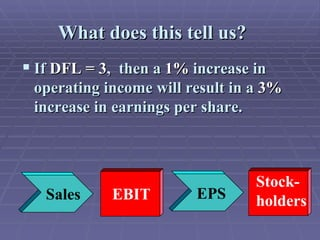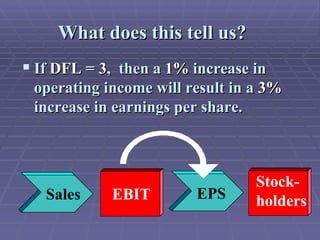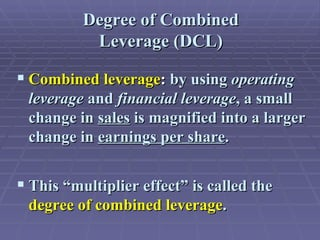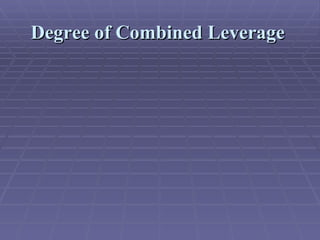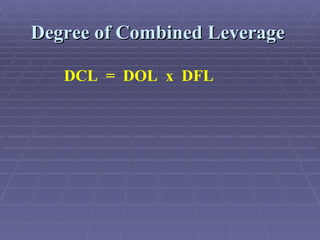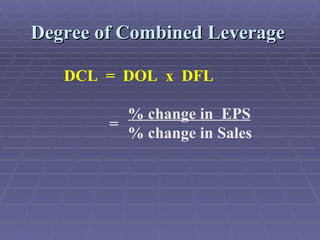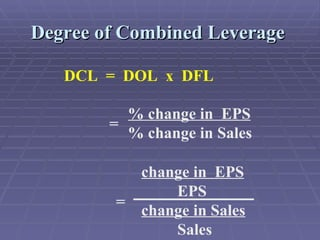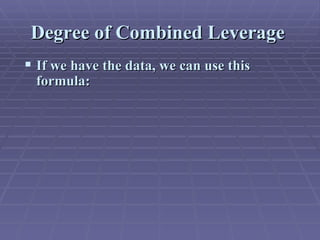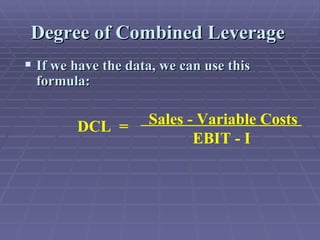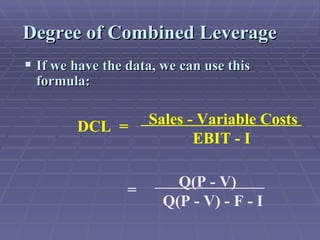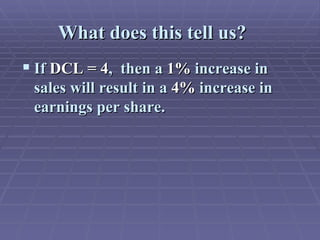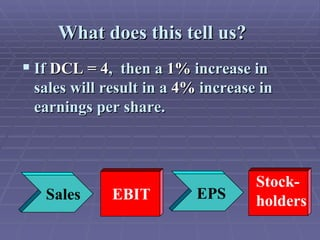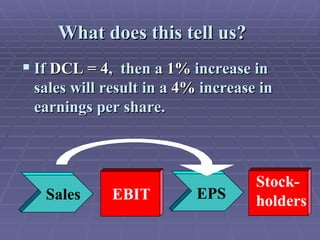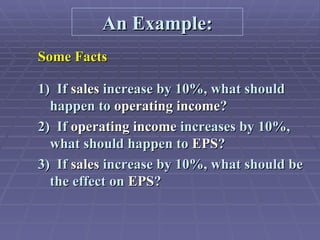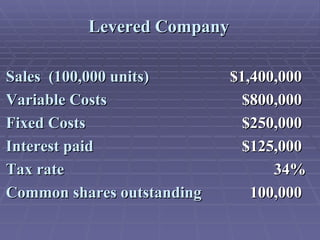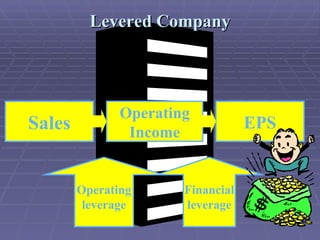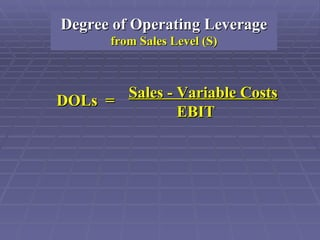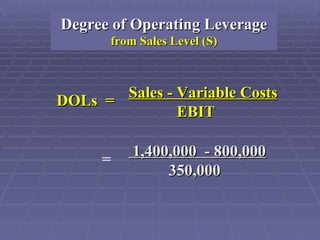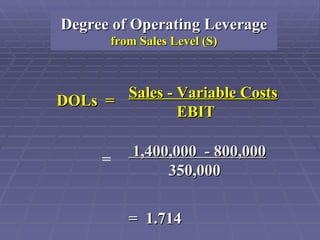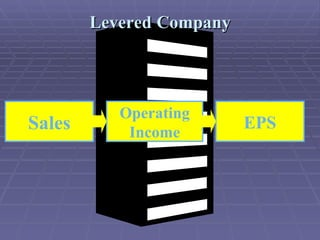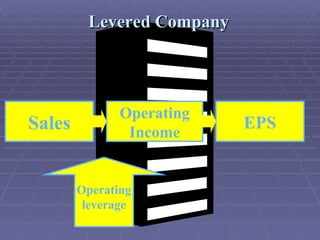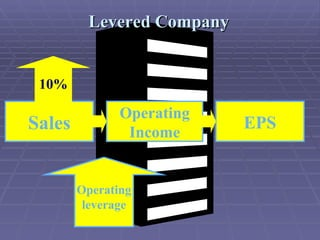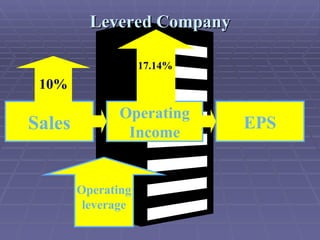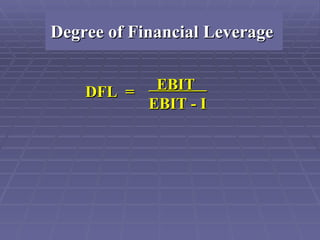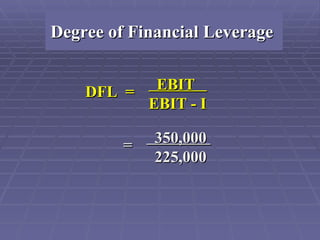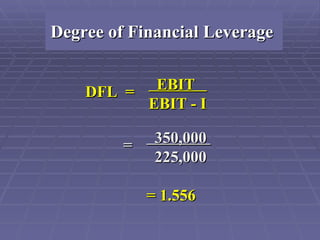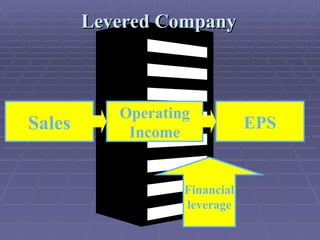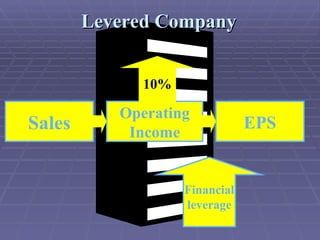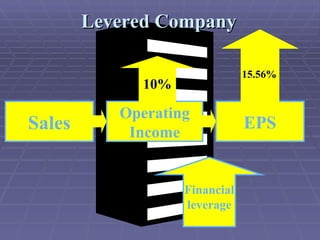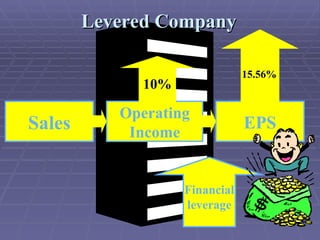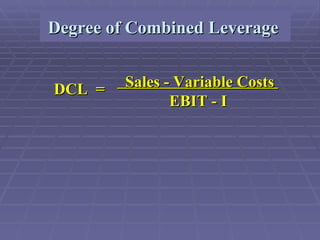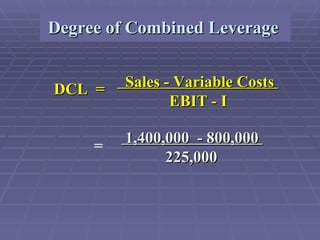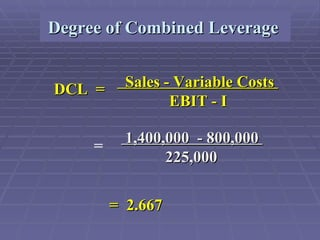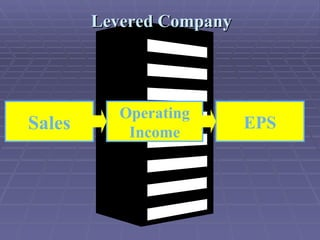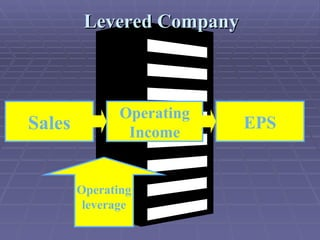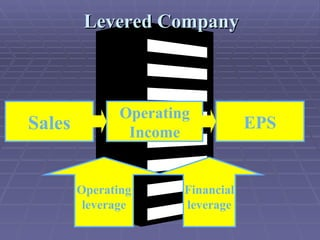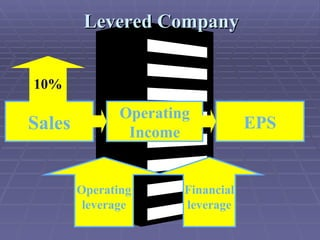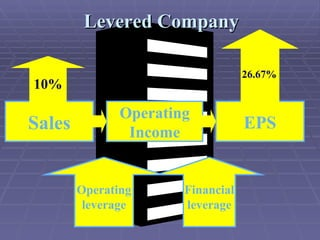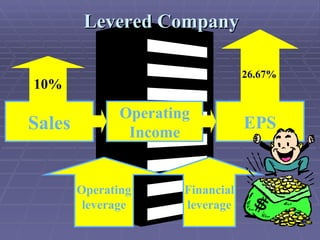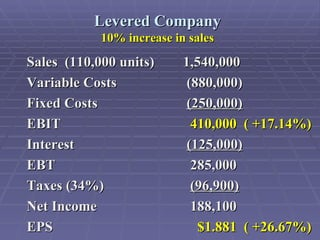1 de 97
Anúncio

### Leverage nikunj

1. What is Leverage?
2. What is Leverage?
3. What is Leverage?
4.
5. EBIT Operating Leverage
6.
7. EPS Financial Leverage
8. Breakeven Analysis Quantity \$
9. Quantity \$ Total Revenue
10. Quantity \$ Total Revenue
11. Quantity { \$ Total Revenue Total Cost FC
12. Quantity { \$ Total Revenue Total Cost FC Q 1 + - } EBIT
13. Quantity { \$ Total Revenue Total Cost FC Break-even point Q 1 + - } EBIT
14. Quantity { \$ Total Revenue Total Cost FC Break- even point Q 1 + - } EBIT
15. Quantity { \$ Total Revenue Total Cost = Fixed FC Break-even point } Q 1 + - EBIT
16. Quantity { \$ Total Revenue Total Cost = Fixed FC Break- even point } Q 1 + - EBIT
17. Trade-off: the firm has a higher breakeven point. If sales are not high enough, the firm will not meet its fixed expenses! Quantity { \$ Total Revenue Total Cost = Fixed FC Break- even point } Q 1 + - EBIT
18. Breakeven Calculations
19. Degree of Operating Leverage from Sales Level (S) DOLs = % change in EBIT % change in sales
20. Degree of Operating Leverage from Sales Level (S) DOLs = % change in EBIT % change in sales change in EBIT EBIT change in sales sales =
21. Degree of Financial Leverage DFL = % change in EPS % change in EBIT
22. Degree of Financial Leverage DFL = % change in EPS % change in EBIT change in EPS EPS change in EBIT EBIT =
23. Degree of Combined Leverage
24. Degree of Combined Leverage DCL = DOL x DFL
25. Degree of Combined Leverage DCL = DOL x DFL % change in EPS % change in Sales =
26. Degree of Combined Leverage DCL = DOL x DFL = % change in EPS % change in Sales change in EPS EPS change in Sales Sales =
27. Levered Company EPS Financial leverage Operating Income Sales Operating leverage
28. Degree of Operating Leverage from Sales Level (S) DOLs = Sales - Variable Costs EBIT
29. Degree of Operating Leverage from Sales Level (S) 1,400,000 - 800,000 350,000 = DOLs = Sales - Variable Costs EBIT
30. Degree of Operating Leverage from Sales Level (S) 1,400,000 - 800,000 350,000 = 1.714 = DOLs = Sales - Variable Costs EBIT
31. Levered Company EPS Operating Income Sales
32. Levered Company EPS Operating Income Sales Operating leverage
33. Levered Company EPS Operating Income Sales Operating leverage 10%
34. Levered Company EPS Operating Income Sales Operating leverage 10% 17.14%
35. Degree of Financial Leverage DFL = EBIT EBIT - I
36. Degree of Financial Leverage DFL = EBIT EBIT - I = 350,000 225,000
37. Degree of Financial Leverage DFL = EBIT EBIT - I = 350,000 225,000 = 1.556
38. Levered Company EPS Operating Income Sales
39. Levered Company EPS Operating Income Sales Financial leverage
40. Levered Company EPS Financial leverage Operating Income Sales 10%
41. Levered Company EPS Financial leverage Operating Income Sales 10% 15.56%
42. Levered Company EPS Financial leverage Operating Income Sales 10% 15.56%
43. Degree of Combined Leverage DCL = Sales - Variable Costs EBIT - I
44. Degree of Combined Leverage DCL = Sales - Variable Costs EBIT - I 1,400,000 - 800,000 225,000 =
45. Degree of Combined Leverage DCL = Sales - Variable Costs EBIT - I 1,400,000 - 800,000 225,000 = 2.667 =
46. Levered Company EPS Operating Income Sales
47. Levered Company EPS Operating Income Sales Operating leverage
48. Levered Company EPS Financial leverage Operating Income Sales Operating leverage
49. Levered Company EPS Financial leverage Operating Income Sales Operating leverage 10%
50. Levered Company EPS Financial leverage Operating Income Sales Operating leverage 10% 26.67%
51. Levered Company EPS Financial leverage Operating Income Sales 10% 26.67% Operating leverage

1. 12
Anúncio